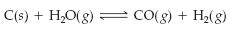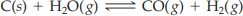×
Get Full Access to Introductory Chemistry - 5 Edition - Chapter 15 - Problem 76p
Get Full Access to Introductory Chemistry - 5 Edition - Chapter 15 - Problem 76p

×

# Solved: Coal can be used to generate hydrogen gas (a potential fuel) by this endothermicISBN: 9780321910295 34

## Solution for problem 76P Chapter 15

Introductory Chemistry | 5th Edition

• Textbook Solutions
• 2901 Step-by-step solutions solved by professors and subject experts
• Get 24/7 help from StudySoup virtual teaching assistantsIntroductory Chemistry | 5th Edition

4 5 1 238 Reviews
27
5
Problem 76P

Problem 76P

Coal can be used to generate hydrogen gas (a potential fuel) by this endothermic reaction.If this reaction mixture is at equilibrium, predict the effect (shift right, shift left, or no effect) of these changes.

(a) adding more C to the reaction mixture

(b) adding more H2O(g) to the reaction mixture

(c) raising the temperature of the reaction mixture

(d) increasing the volume of the reaction mixture

(e) adding a catalyst to the reaction mixture

Step-by-Step Solution:

Problem 76P

Coal can be used to generate hydrogen gas (a potential fuel) by this endothermic reaction.If this reaction mixture is at equilibrium, predict the effect (shift right, shift left, or no effect) of these changes.

(a) adding more C to the reaction mixture

(b) adding more H2O(g) to the reaction mixture

(c) raising the temperature of the reaction mixture

(d) increasing the volume of the reaction mixture

(e) adding a catalyst to the reaction mixture

Step by Step Solution

Step 1 of 5

(a)

­­­­According to Le Chatelier’s principle “When a chemical system at equilibrium is disturbed, the system shifts in a direction that minimizes the disturbance.

The given reaction is as follows.The given – Adding more “C” to the reaction mixture.

The Carbon element is present in the solid state and it is one of the reactants in the given reaction mixture. Solid state compounds don't affect the equilibrium of the reaction.

Therefore, there is no effect for adding more “C” to the reaction mixture.

_________________________________________________________________________

Step 2 of 5

Step 3 of 5

##### ISBN: 9780321910295

Since the solution to 76P from 15 chapter was answered, more than 355 students have viewed the full step-by-step answer. The full step-by-step solution to problem: 76P from chapter: 15 was answered by , our top Chemistry solution expert on 05/06/17, 06:45PM. The answer to “Coal can be used to generate hydrogen gas (a potential fuel) by this endothermic reaction. If this reaction mixture is at equilibrium, predict the effect (shift right, shift left, or no effect) of these changes.(a) adding more C to the reaction mixture(b) adding more H2O(g) to the reaction mixture(c) raising the temperature of the reaction mixture(d) increasing the volume of the reaction mixture(e) adding a catalyst to the reaction mixture” is broken down into a number of easy to follow steps, and 70 words. Introductory Chemistry was written by and is associated to the ISBN: 9780321910295. This textbook survival guide was created for the textbook: Introductory Chemistry, edition: 5. This full solution covers the following key subjects: reaction, mixture, adding, shift, effect. This expansive textbook survival guide covers 19 chapters, and 2046 solutions.

Unlock Textbook Solution